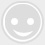【施工技术】现浇连续梁桥支架搭设及预压施工技术控制

-回复 -浏览1、技术准备

2、试验准备

3、测量准备

1、边跨现浇段支架受力分析

1.1 荷载计算

1）箱梁砼自重：q=11.352*3*9.8/12=27.812 KN/m2。

2）模板及附件重统一取：q=3.5 KN/m2。

3）施工活载取：q=3 KN/m2。

4）钢管自重q：q=520/（7.5*12）=5.78 kN/m2

∑q=Q=K（q+q+q+q）=1.5×（27.812+3.5+3.0+5.78）=60.138 kN/m2

1.2 立杆强度验算

1）立杆承受荷载：

N=QA=60.138×0.6×0.6=21.65 KN。

2）立杆稳定验算：N/φA≤[fc]

[fc]―钢管设计强度，[fc]=205 N/mm2；
φ―立杆稳定系数；
A―钢管截面积，A=489 mm2；
N―立杆承受的竖向力，N=21.65 KN；
i―钢管回转半径，i=15.8 mm≥0.402。
λ=987.5/i=62.5 查表得φ=0.784>0.402 稳定。

fc=37.51 N/mm2<[fc]=205 N/mm2。

fc=75.02 N/mm2<[fc]=205 N/mm2 立杆稳定。

1.3 横向杆稳定验算

q=q+q=1.5+0.0247=1.52 KN/m2

q为施工人群荷载。

[fc]―钢管设计抗弯强度，[fc]=205 KN/mm2；

W―钢管截面抵抗矩， W=5 078 mm3；

[M]=205×5 078=1 040 990 N・mm=1.041 KN・m
Mmax=0.456 KN・m<[M]=1.041 KN・m

1.4 支架刚度（挠度）验算

ωmax―最大挠度；
E―钢管弹性模量，E=2.05×105 N/mm2；
q―均布荷载， q=1.52 KN/m=1.52 N/mm；
I―钢管截面抵抗矩，I=12.19 cm4=12.19×104 mm4。
ωmax=0.103 mm

1.5 纵向大枋强度验算

I=15*10*10*10/12=1 250 cm4

W=10*15*15/6=375 cm3

1）方木抗弯强度计算：

2）方木抗剪强度计算：

3）方木挠度计算：

1.6 横枋强度验算

2、总结论

1、预压荷载的计算

1）翼缘板：

2）腹板及底板：

2、 预压袋重量的确定

3、预压每延米预压袋的袋数

1）腹板及底板宽6.7 m范围内5.23/1.2=4.4袋/m2；

2）翼缘板处1.577/1.2=1.3袋/m2。

4、预压观测点点位布置

5、预压

6、变形观测点的数据处理

（来源：路桥网）